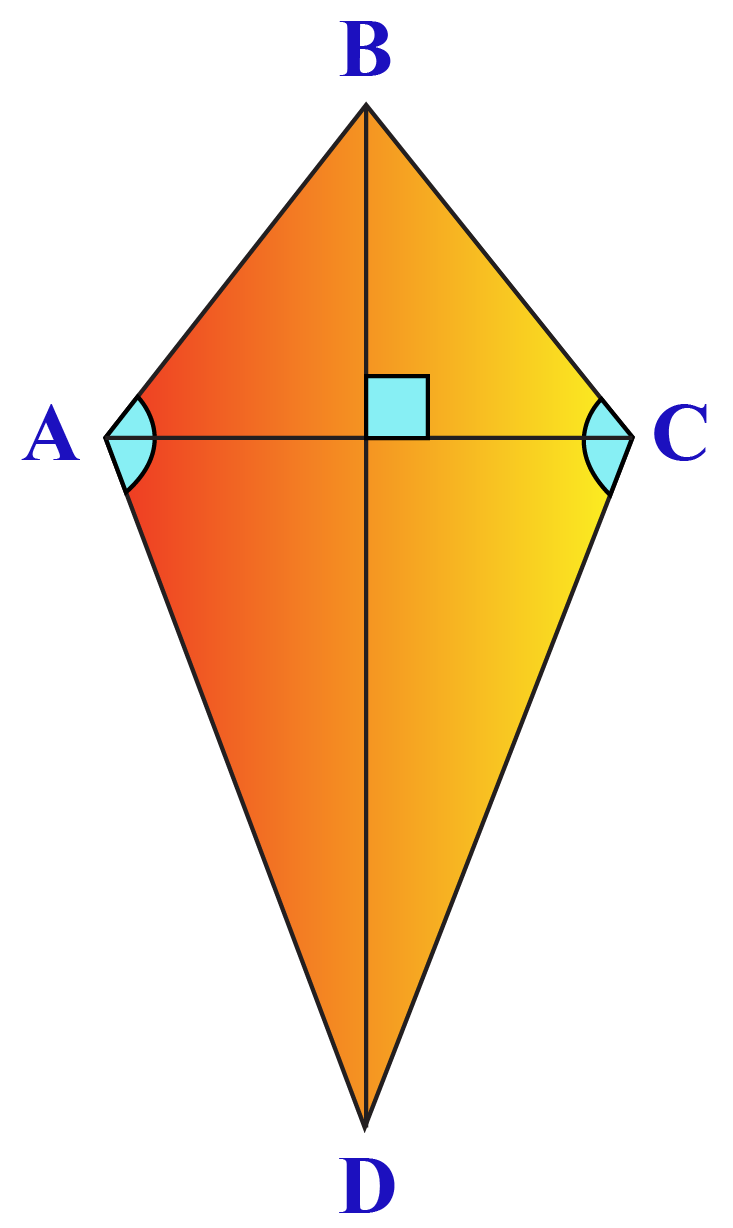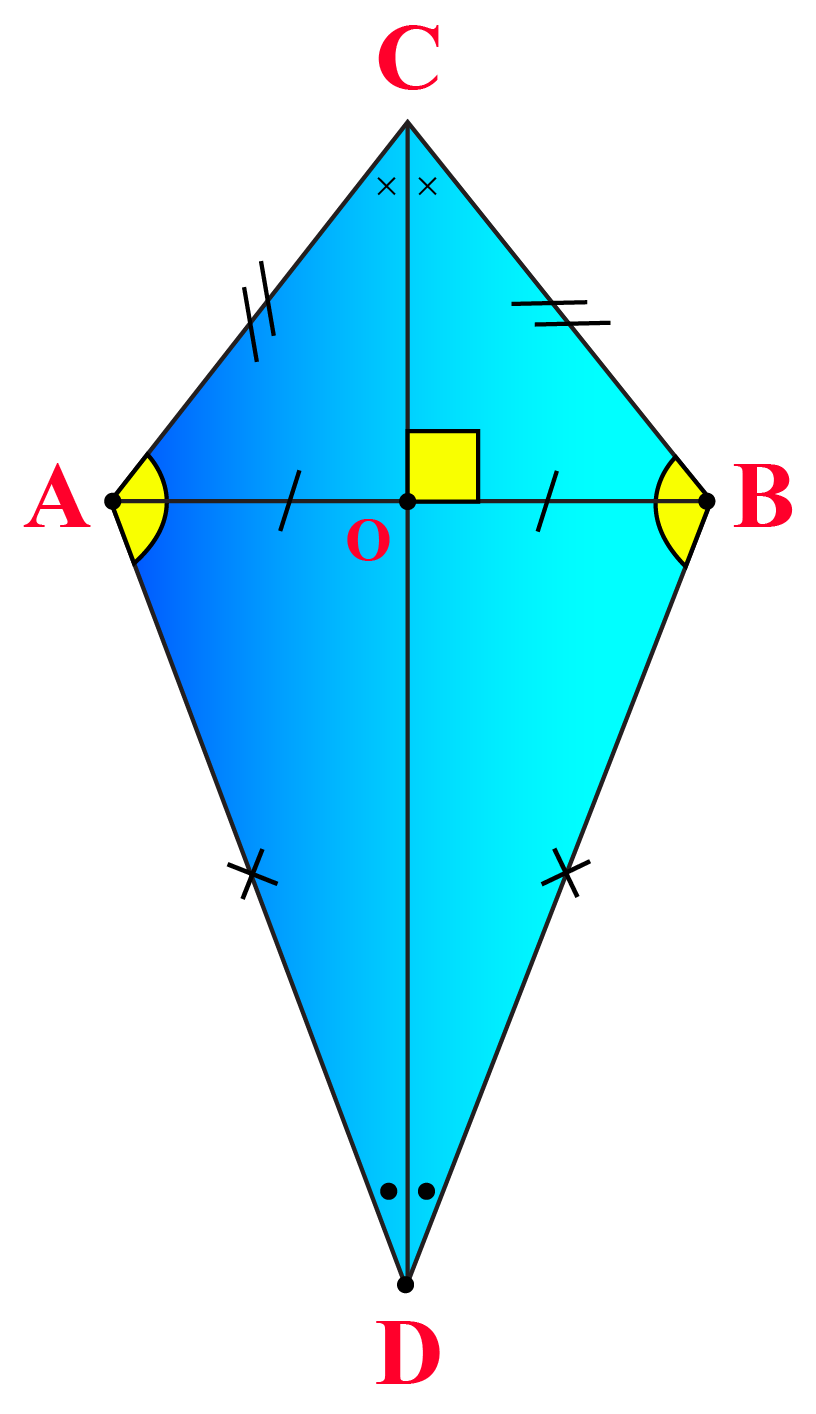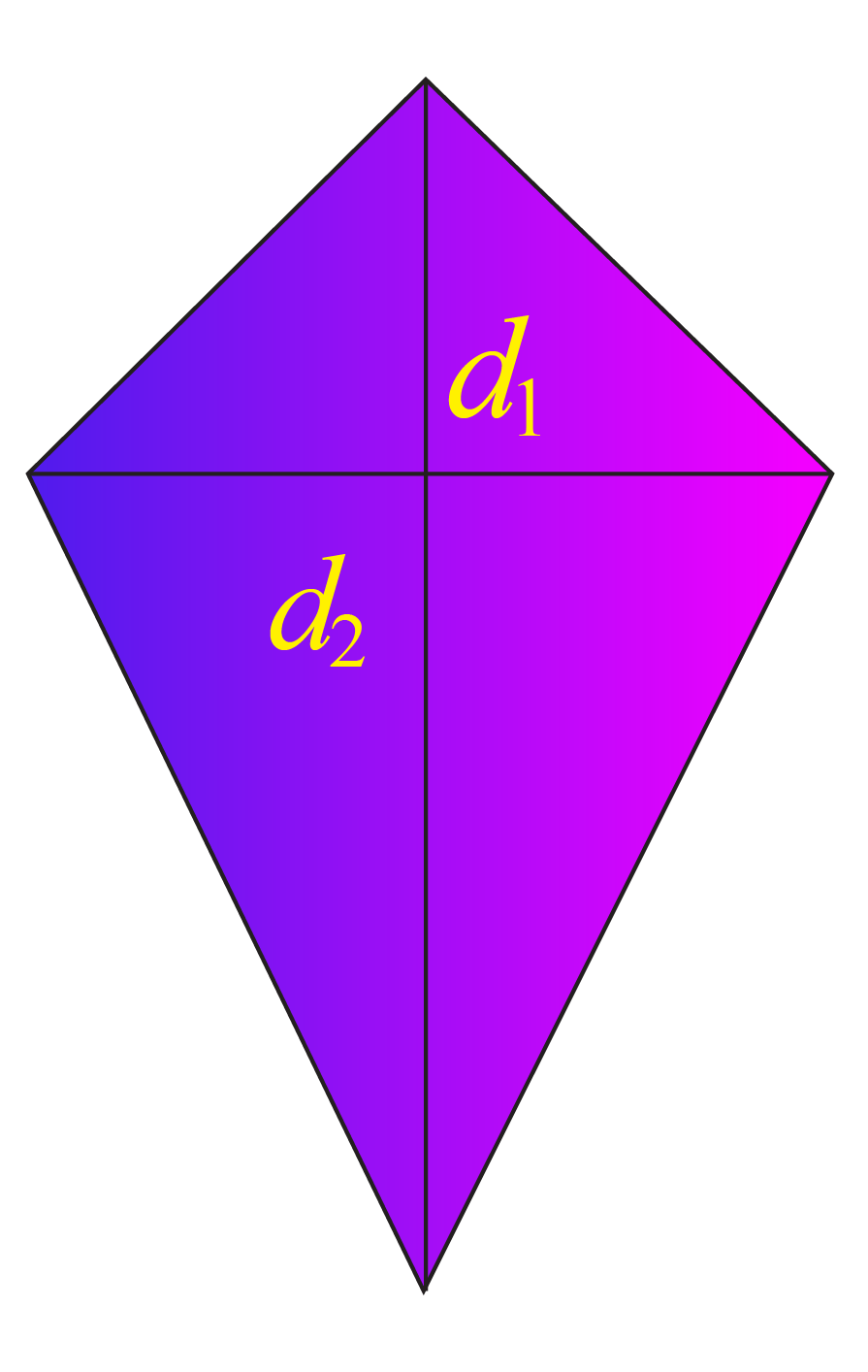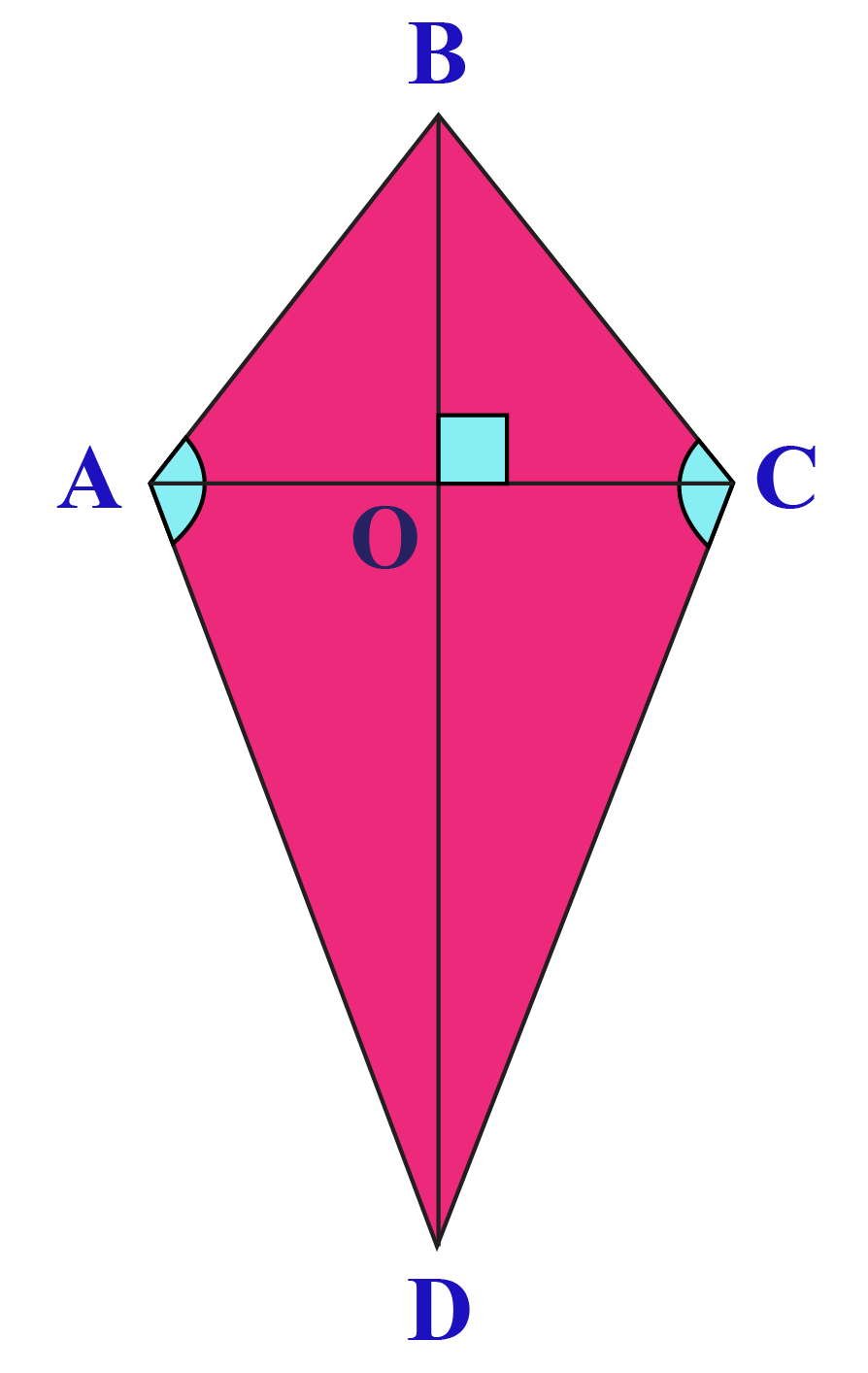# Area of a kite

Area of a kite
Go back to  'Area'

In this mini-lesson, we will explore the world of kites.

You will get to learn about the interesting concepts related to the definition of a kite, its properties, and the area of a kite.

A closed figure made with 4 line segments forms the shape of a kite.

We will explore everything about kites, which are commonly seen around us.

You can observe the shape of a kite in the kites flown by kids in the sky, and an Indian dessert - Kaju Katli.So let's get started!

## What Is a Kite and its Properties?

A kite is a quadrilateral in which two pairs of adjacent sides are equal.

The elements of a kite are its 4 angles, its 4 sides, and 2 diagonals.

Let's look at the kite ABCD.The elements of this kite are:

• $$\angle ABC$$
• $$\angle BCD$$
• $$\angle CDA$$
• $$\angle DAB$$
• Side AB
• Side BC
• Side CD
• Diagonal AC
• Diagonal BD

### Properties of KiteA kite has:

• Two pairs of adjacent equal sides

$$AC = BC \text { and } AD = BD$$

• One pair of opposite angles (which are obtuse) that are equal

$$\angle A = \angle B$$

• Diagonals that are perpendicular to each other

$$AB \perp CD$$

• A longer diagonal that bisects the shorter diagonal

$$AO=OB$$

• a longer diagonal that bisects the pair of opposite angles

$$\angle ACD = \angle DCB$$

$$\angle ADC = \angle CDB$$

## What Is the Area of a Kite?

###The area of a kite is half the product of the lengths of its diagonals.

The formula to determine the area of a kite is:

 Area = $$\dfrac{1}{2}\times d_1 \times d_2$$

The area of kite ABCD given below is $$\dfrac{1}{2}\times AC \times BD$$## Unlock the Derivation of the Formula

Consider a kite ABCD as shown above.

Assume the lengths of the diagonals of ABCD to be $$AC = p, BD = q$$

We know that the longer diagonal of a kite bisects the shorter diagonal at right angles, i.e., BD bisects AC and $$\angle AOB = 90^\circ, \angle BOC = 90^\circ$$

Therefore,

$$AO=OC=\dfrac{AC}{2}=\dfrac{p}{2}$$

Area of kite $$ABCD$$ = Area of $$\Delta ABD$$ + Area of $$\Delta BCD\ \ \ \ \cdots (1)$$

We know that,

Area of a triangle = $$\dfrac{1}{2}\times \text{Base}\times \text{Height}$$

Now, we will calculate the areas of triangles ABD and BCD

Area of $$\Delta ABD = \dfrac{1}{2}\times AO \times BD$$ = $$\dfrac{1}{2}\times \dfrac{p}{2}\times q=\dfrac{pq}{4}$$

Area of $$\Delta BCD = \dfrac{1}{2}\times OC \times BD$$ =$$\dfrac{1}{2}\times \dfrac{p}{2}\times q=\dfrac{pq}{4}$$

Therefore, using (1)

\begin{align} \text{Area of kite ABCD }&= \dfrac{pq}{4}+\dfrac{pq}{4}\\[0.2 cm]&=\dfrac{pq}{2}\\[0.2 cm]&=\dfrac{1}{2}\times BD\times AC \end{align}

## How Do you Calculate the Area of a Kite?

The area of a kite is calculated by plugging in the lengths of its diagonals into the formula of the area of the kite.

Enter the lengths of the diagonals of a kite and hit the 'Calculate' button to determine its area.

More Important Topics
Numbers
Algebra
Geometry
Measurement
Money
Data
Trigonometry
Calculus